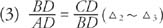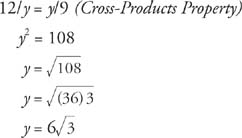## Altitude to the Hypotenuse

In Figure 1, right triangle ABC has altitude BD drawn to the hypotenuse AC.Figure 1 An altitude drawn to the hypotenuse of a right triangle.

The following theorem can now be easily shown using the AA Similarity Postulate.

Theorem 62: The altitude drawn to the hypotenuse of a right triangle creates two similar right triangles, each similar to the original right triangle and similar to each other.

Figure 2 shows the three right triangles created in Figure . They have been drawn in such a way that corresponding parts are easily recognized.Figure 2 Three similar right triangles from Figure (not drawn to scale).

Note that AB and BC are legs of the original right triangle; AC is the hypotenuse in the original right triangle; BD is the altitude drawn to the hypotenuse; AD is the segment on the hypotenuse touching leg AB and DC is the segment on the hypotenuse touching leg BC.

Because the triangles are similar to one another, ratios of all pairs of corresponding sides are equal. This produces three proportions involving geometric means.These two proportions can now be stated as a theorem.

Theorem 63: If an altitude is drawn to the hypotenuse of a right triangle, then each leg is the geometric mean between the hypotenuse and its touching segment on the hypotenuse.This proportion can now be stated as a theorem.

Theorem 64: If an altitude is drawn to the hypotenuse of a right triangle, then it is the geometric mean between the segments on the hypotenuse.

Example 1: Use Figure 3 to write three proportions involving geometric means.Figure 3 Using geometric means to write three proportions.Example 2: Find the values for x and y in Figures 4 (a) through (d).Figure 4 Using geometric means to find unknown parts.

Because it represents a length, x cannot be negative, so x = 12.

By Theorem 63xy = y/9

Because x = 12, from earlier in the problem,